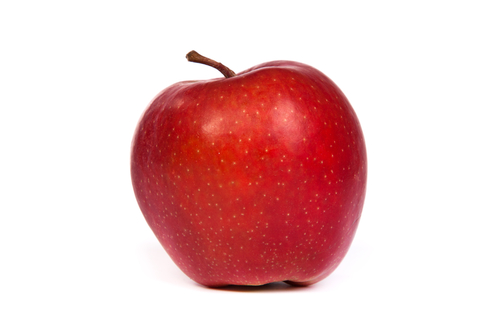#Teacher Note: Names on either side of =¶

Notice that we can use a variable name on either side of the equal sign, = (the assignment operator). However, also notice that a variable name is treated differently when found on the left or the right of the equal sign .

For instance, consider this series of assignment statements:

If we think of a variable as a box that holds a value, the meaning of line 1 is fairly obvious: place the value 5 in the box labelled a. In line 1, a stands for the box associated with the name a.

In line 2, however, a is found on the right side of = . In this case, a no longer stands for the box labelled a , but rather, the value stored in the box labelled a . Therefore, a + 1 in this case actually means 5 + 1 . In fact, line 2 is executed in these steps:

1. Evaluate the expression a + 1 to produce a value

2. Replace a in the expression with the value stored in a, to produce the expression 5 + 1

3. Evaluate the expression 5 + 1 to produce the value 6

4. Store the value produced (6) in the variable b

Of course, the computer performs all these steps for you!

After the first two lines are executed, the print statements will show that a will contain 5, while b will contain 6.

## Incrementing a variable¶

It’s important to understand the above steps to make sense of the following example. This is a common operation in which the value of a variable is incremented (it’s value is increased by 1):

Line 1 may be straightforward to interpret (assign 5 to the box labelled a ), but in line 2, a appears twice! This may prove to be confusing to some students. But armed with the above understanding, we can interpret line 2 as follows:

1. Evaluate the expression a + 1 to produce a value

2. Replace a in the expression with the value stored in a, to produce the expression 5 + 1

3. Evaluate the expression 5 + 1 to produce the value 6

4. Store the value produced (6) in the variable a

Run the above code to see what the value of the box labelled a is when it is printed.

### Self check¶

Consider the following code when trying to solve the problem below:

distance = 924.7
mpg = 35.5
gallons = distance / mpg


The following mixed-up blocks represent the steps that the computer performs to execute these lines. Drag the blocks from the left and put them in the correct order on the right. Click the <i>Check Me</i> button to check your solution.</p>